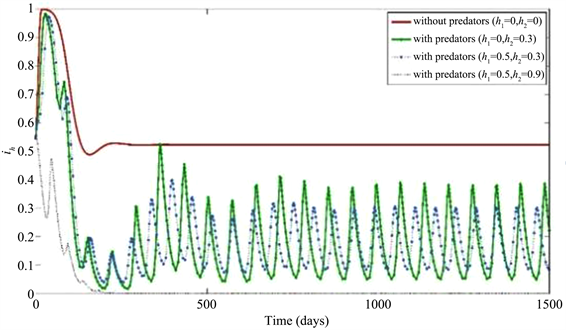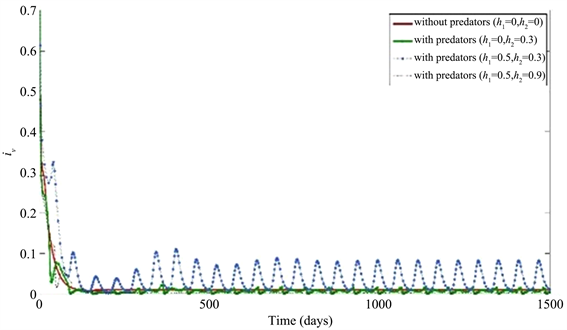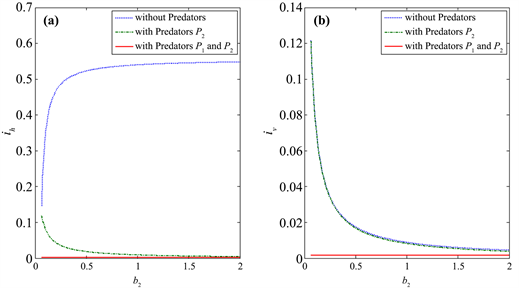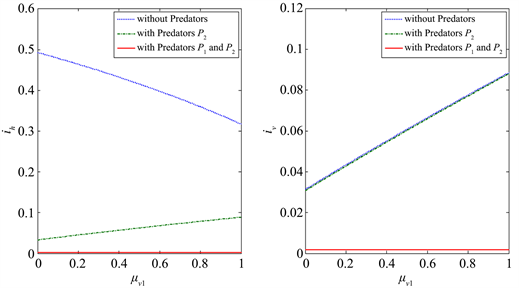﻿ 多天敌作用下的阶段结构虫媒传染病动力学

# 多天敌作用下的阶段结构虫媒传染病动力学Multi-Predator Effects on the Host-Vector Disease Dynamics with Stage Structure

Abstract: In this paper, we study the dynamics of vector-borne disease model with stage structure under the impact of Larval natural enemies and adult natural enemies. Based on numerical simulation, the effects of vector-borne birth rate, death rate and conversion rate on the epidemic area and equilibrium value were analyzed to provide theoretical basis for long-term control of vector-borne disease.

1. 引言

2. 主要内容

2.1. 模型

$\left\{\begin{array}{l}\frac{\text{d}{S}_{h}\left(t\right)}{\text{d}t}={b}_{1}-{\beta }_{1}{S}_{h}\left(t\right){I}_{v}\left(t\right)-{\mu }_{h}{S}_{h}\left(t\right),\\ \frac{\text{d}{I}_{h}\left(t\right)}{\text{d}t}={\beta }_{1}{S}_{h}\left(t\right){I}_{v}\left(t\right)-\left({\mu }_{h}+{\delta }_{h}\right){I}_{h}\left(t\right),\\ \frac{\text{d}{M}_{v}\left(t\right)}{\text{d}t}={b}_{2}\left({S}_{v}\left(t\right)+{I}_{v}\left(t\right)\right)-\left({\mu }_{v1}+d\right){M}_{v}\left(t\right)-\frac{{h}_{1}{M}_{v}\left(t\right){P}_{1}\left(t\right)}{1+{a}_{1}{M}_{v}\left(t\right)},\\ \frac{\text{d}{P}_{1}\left(t\right)}{\text{d}t}=\frac{{\gamma }_{1}{h}_{1}{M}_{v}\left(t\right){P}_{1}\left(t\right)}{1+{a}_{1}{M}_{v}\left(t\right)}-{e}_{1}{P}_{1}\left(t\right),\\ \frac{\text{d}{S}_{v}\left(t\right)}{\text{d}t}=d{M}_{v}\left(t\right)-{\beta }_{2}{S}_{v}\left(t\right){I}_{h}\left(t\right)-{\mu }_{v2}{S}_{v}\left(t\right)-\alpha {S}_{v}\left(t\right)\left({S}_{v}\left(t\right)+{I}_{v}\left(t\right)\right)-\frac{{h}_{2}{S}_{v}\left(t\right){P}_{2}\left(t\right)}{1+{a}_{2}{N}_{v}\left(t\right)},\\ \frac{\text{d}{I}_{v}\left(t\right)}{dt}={\beta }_{2}{S}_{v}\left(t\right){I}_{h}\left(t\right)-{\mu }_{v2}{I}_{v}\left(t\right)-\alpha {I}_{v}\left(t\right)\left({S}_{v}\left(t\right)+{I}_{v}\left(t\right)\right)-\frac{{h}_{2}{I}_{v}\left(t\right){P}_{2}\left(t\right)}{1+{a}_{2}{N}_{v}\left(t\right)},\\ \frac{\text{d}{P}_{2}\left(t\right)}{\text{d}t}=\frac{{\gamma }_{2}{h}_{2}{N}_{v}\left(t\right){P}_{2}\left(t\right)}{1+{a}_{2}{N}_{v}\left(t\right)}-{e}_{2}{P}_{2}\left(t\right),\end{array}$ (1)

${R}_{0}={b}_{1}{\beta }_{1}{\beta }_{2}{\stackrel{˜}{S}}_{v}/\left[{\mu }_{h}\left({\mu }_{h}+{\delta }_{h}\right)\left({\mu }_{v2}+\alpha {\stackrel{˜}{S}}_{v}+\frac{{h}_{2}{\stackrel{˜}{P}}_{2}}{1+{a}_{2}{\stackrel{˜}{S}}_{v}}\right)\right],$

${\stackrel{˜}{P}}_{2}=\left(1+{a}_{2}{\stackrel{˜}{S}}_{v}\right)\left[d{\stackrel{˜}{M}}_{v}-{\mu }_{v2}{\stackrel{˜}{S}}_{v}-\alpha {\stackrel{˜}{S}}_{v}^{2}\right]/\left({h}_{2}{\stackrel{˜}{S}}_{v}\right),{\stackrel{˜}{M}}_{v}={e}_{1}/\left({\gamma }_{1}{h}_{1}-{a}_{1}{e}_{1}\right),{\stackrel{˜}{S}}_{v}={e}_{2}/\left({\gamma }_{2}{h}_{2}-{a}_{2}{e}_{2}\right).$

2.2. 多天敌策略分析

${i}_{h}\left(t\right)=\frac{t\text{ }时刻的染病宿主量}{t\text{ }时刻的宿主总量}=\frac{{I}_{h}\left(t\right)}{{N}_{h}\left(t\right)},{i}_{v}\left(t\right)=\frac{t\text{ }时刻的染病虫媒量}{t\text{ }时刻的虫媒总量}=\frac{{I}_{v}\left(t\right)}{{N}_{v}\left(t\right)}.$

${b}_{1}=0.003,{\mu }_{h}=0.014/365,{\delta }_{h}=18.25/365,{\beta }_{1}=14.1/365,{\mu }_{v}=0.014/365,$

$d=0.5,{\beta }_{2}=49.35/365,\alpha =0.005,{a}_{1}=0.1,{\gamma }_{1}=0.1,{e}_{1}=0.1,{a}_{2}=0.1,{\gamma }_{2}=0.15,{e}_{2}=0.15$

1) ih和iv关于不同天敌捕获率的仿真结果

2) 阶段结构因素对虫媒疾病传播的影响Figure 1. The population density of infected hosts with no natural enemies, single natural enemies and multiple natural enemiesFigure 2. The population density of infected vectors with no natural enemies, single natural enemies and multiple natural enemiesFigure 3. The change of disease prevalence ih and iv with larval birth rate b2of the immature vectorsFigure 4. The change of disease prevalence ih and iv with larval conversation rate d of the immature vectorsFigure 5. The change of disease prevalence ih and iv with larval death rate μv1 of the immature vectors

3. 小结

 杨希, 黄金水, 何学友, 等. 管氏肿腿蜂及其带菌室内防治松墨天牛幼虫试验[J]. 福建林业科技, 2005, 32(3): 94-99.

 刘洪剑, 朴春根, 汪来发, 等. 白僵菌和肿腿蜂对松墨天牛幼虫的作用[J]. 林业科学, 2007, 43(5): 64-68.

 Zhou, F.Y. Ma, C.R., Liang, H.Z., Dai, B.X. and Yao, H.X. (2018) Impact of Predator on the Host-Vector Disease Model with Stage Structure for the Vector. Advances in Difference Equations, 324, 1-22.
https://doi.org/10.1186/s13662-018-1777-6

 Diekmann, O., Heesterbeek, J.A.P. and Metz, J.A.J. (1990) On the Definition and the Computation of the Basic Reproduction Ratio R0 in Models for Infectious Diseases in Heterogeneous Populations. Journal of Mathematical Biology, 28, 365-382.
https://doi.org/10.1007/BF00178324

 Driessche, V.P. and Watmough, J. (2002) Reproduction Numbers and Sub-Threshold Endemic Equilibria for Compartmental Models of Disease Transmission. Mathematical Biosciences, 180, 29-48.
https://doi.org/10.1016/S0025-5564(02)00108-6

 Moore, S.M., Borer, E.T. and Hosseini, P.R. (2009) Predators Indirectly Control Vector-Borne Disease: Linking Predator-Prey and Host-Pathogen Models. Journal of the Royal Society Interface, 7, 161-176.
https://doi.org/10.1098/rsif.2009.0131

 Zhou, F.Y. and Yao, H.X. (2014) Dynamics and Biocontrol: The Indirect Effects of a Predator Population on a Host-Vector Disease Model. Abstract and Applied Analysis, 2014, Article ID 252718.
https://doi.org/10.1155/2014/252718

Top